Colin Maclaurin (1698 - 1746)

From A Short Account of the History of Mathematics' (4th edition, 1908) by W. W. Rouse Ball.

Colin Maclaurin, who was born in Kilmodan in Argyllshire in February 1698, and died at York on June 14; 1746, was educated at the university of Glasgow; in 1717 he was elected, at the early age of nineteen, professor of mathematics at Aberdeen; and in 1725 he was appointed the deputy of the mathematical professor at Edinburgh, and ultimately succeeded him. There was some difficulty in securing a stipend for a deputy, and Newton privately wrote offering to bear the cost so as to enable the university to secure the services of Maclaurin. Maclaurin took an active part in opposing the advance of the Young Pretender in 1745; on the approach of the Highlanders he fled to York, but the exposure in the trenches at Edinburgh and the privations he endured in his escape proved fatal to him.

His chief works are his Geometria Organica, London, 1720; his De Linearum Geometricarum Proprietatibus, London, 1720; his Treatise on Fluxions, Edinburgh, 1742; his Algebra, London, 1748; and his Account of Newton's Discoveries, London, 1748.

The first section of the first part of the Geometria Organica is on conics; the second on nodal cubics; the third on other cubics and on quartics; and the fourth section is on general properties of curves. Newton had shewn that, if two angles bounded by straight lines turn round their respective summits so that the point of intersection of two of these lines moves along a straight line, the other point of intersection will describe a conic; and, if the first point move along a conic, the second will describe a quartic. Maclaurin gave an analytical discussion of the general theorem, and shewed how by this method various curves could be practically traced. This work contains an elaborate discussion on curves and their pedals, a branch of geometry which he had created in two papers published in the Philosophical Transactions for 1718 and 1719.

The second part of the work is divided into three sections and an appendix. The first section contains a proof of Cotes's theorem above alluded to; and also the analogous theorem (discovered by himself) that, if a straight line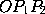... drawn through a fixed point O cut a curve of the nth degree in n points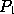,,..., and if the tangents at,,... cut a fixed line Ox in points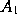,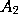,..., then the sum of the reciprocals of the distances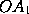,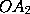,... is constant for all positions of the line.... These two theorems are generalizations of those given by Newton on diameters and asymptotes. Either is deducible from the other. In the second and third sections these theorems are applied to conics and cubics; most of the harmonic properties connected with a quadrilateral inscribed in a conic are determined; and in particular the theorem on an inscribed hexagon which is known by the name of Pascal is deduced. Pascal's essay was not published till 1779, and the earliest printed enunciation of this theorem was that given by Maclaurin. Amongst other propositions he shews that, if a quadrilateral be inscribed in a cubic, and if the points of intersection of the opposite sides also lie on the curve, then the tangents to the cubic at any two opposite angles of the quadrilateral will meet on the curve. In the fourth section he considers some theorems on central force. The fifth section contains some theorems on the description of curves through given points. One of these (which includes Pascal's as a particular case) is that if a polygon be deformed so that while each of its sides passes through a fixed point its angles (save one) describe respectively curves of the mth, nth, pth,... degrees, then shall a remaining angle describe a curve of the degree 2mnp...; but if the given points be collinear, the resulting curve will be only of the degree mnp.... This essay was reprinted with additions in the Philosophical Transactions for 1735.

The Treatise of Fluxions, published in 1742, was the first logical and systematic exposition of the method of fluxions. The cause of its publication was an attack by Berkeley on the principles of the infinitesimal calculus, In it [art. 751, p.610] Maclaurin gave a proof of the theorem that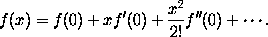This was obtained in the manner given in many modern textbooks by assuming that f(x) can be expanded in a form like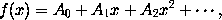then, on differentiating and putting x = 0 in the successive results, the values of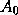,,... are obtained; but he did not investigate the convergency of the series. The result had been previously given in 1730 by James Stirling in his Methodus Differentialis [p. 102], and of course it is at once deducible from Taylor's Theorem. Maclaurin also here enunciated [art. 350, p. 289] the important theorem that, if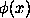be positive and decrease as x increases from x = a to x =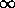, then the series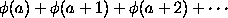is convergent or divergent as the integral from x = a to x =ofis finite or infinite. The theorem had been given by Euler in 1732, but in so awkward a form that its value escaped general attention. Maclaurin here also gave the correct theory of maxima and minima, and rules for finding and discriminating multiple points.

This treatise is, however, especially valuable for the solutions it contains of numerous problems in geometry, statics, the theory of attractions, and astronomy. To solve these Maclaurin reverted to classical methods, and so powerful did these processes seem, when used by him, that Clairaut, after reading the work, abandoned analysis, and attacked the problem of the figure of the earth again by pure geometry. At a later time this part of the book was described by Lagrange as the `chef d'oeuvre de géométrie qu'on peut comparer à tout ce qu'Archimède nous a laissé de plus beau et de plus ingénieux.'' Maclaurin also determined the attraction of a homogeneous ellipsoid at an internal point, and gave some theorems on its attraction at an external point; in attacking these questions he introduced the conception of level surfaces, that is, surfaces, at every point of which the resultant attraction is perpendicular to the surface. No further advance in the theory of attractions was made until Lagrange in 1773 introduced the idea of the potential. Maclaurin also shewed that a spheroid was a possible form of equilibrium of a mass of homogeneous liquid rotating about an axis passing through its centre of mass. Finally he discussed the tides; this part had been previously published (in 1740) and had received a prize from the French Academy.

Among Maclaurin's minor works is his Algebra, published in 1748, and founded on Newton's Universal Arithmetic. It contains the results of some early papers of Maclaurin; notably of two, written in 1726 and 1729, on the number of imaginary roots of an equation, suggested by Newton's theorem; and of one, written in 1729, containing the well-known rule for finding equal roots by means of the derived equation. In this book negative quantities are treated as being not less real than positive quantities. To this work a treatise, entitled De Linearum Geometricarum Proprietatibus Generalibus, was added as an appendix; besides a paper of 1720 above alluded to, it contains some additional and elegant theorems. Maclaurin also produced in 1728 an exposition of the Newtonian philosophy, which is incorporated in the posthumous work printed in 1748. Almost the last paper he wrote was one printed in the Philosophical Transactions for 1743 in which he discussed from a mathematical point of view the form of a bee's cell.

Maclaurin was one of the most able mathematicians of the eighteenth century, but his influence on the progress of British mathematics was on the whole unfortunate. By himself abandoning the use both of analysis and of the infinitesimal calculus, he induced Newton's countrymen to confine themselves to Newton's methods, and it was not until about 1820, when the differential calculus was introduced into the Cambridge curriculum, that English mathematicians made any general use of the more powerful methods of modern analysis.

This page is included in a collection of mathematical biographies taken from A Short Account of the History of Mathematics by W. W. Rouse Ball (4th Edition, 1908).

Transcribed by

D.R. Wilkins
(dwilkins@maths.tcd.ie)
School of Mathematics
Trinity College, Dublin